1617973320

# ValueError: invalid literal for int() with base 10: ''

Python throws the error, ValueError: invalid literal for int() with base 10:”, when you pass a float string in `int()` function. Although, you can pass the string in `float()` function.

Consider this example –

``````>>> int('5555.00')
Traceback (most recent call last):
File "<stdin>", line 1, in <module>
ValueError: invalid literal for int() with base 10: '5555.00'
``````

PythonCopy

The problem with this code is that you are passing a float string `'5555.00'` in int() function. The correct way of doing this is to first convert it into `float()` and then into `int()`.

#python #error #python error #python-short

## Buddha Community1666082925

## How to Create Arrays in Python

### In this tutorial, you'll know the basics of how to create arrays in Python using the array module. Learn how to use Python arrays. You'll see how to define them and the different methods commonly used for performing operations on them.

This tutorialvideo on 'Arrays in Python' will help you establish a strong hold on all the fundamentals in python programming language. Below are the topics covered in this video:
1:15 What is an array?
2:53 Is python list same as an array?
3:48  How to create arrays in python?
7:19 Accessing array elements
9:59 Basic array operations
- 10:33  Finding the length of an array
- 15:06  Removing elements
- 18:32  Array concatenation
- 20:59  Slicing
- 23:26  Looping

Python Array Tutorial – Define, Index, Methods

In this article, you'll learn how to use Python arrays. You'll see how to define them and the different methods commonly used for performing operations on them.

The artcile covers arrays that you create by importing the `array module`. We won't cover NumPy arrays here.

1. Introduction to Arrays
1. The differences between Lists and Arrays
2. When to use arrays
2. How to use arrays
1. Define arrays
2. Find the length of arrays
3. Array indexing
4. Search through arrays
5. Loop through arrays
6. Slice an array
3. Array methods for performing operations
1. Change an existing value
3. Remove a value
4. Conclusion

Let's get started!

## What are Python Arrays?

Arrays are a fundamental data structure, and an important part of most programming languages. In Python, they are containers which are able to store more than one item at the same time.

Specifically, they are an ordered collection of elements with every value being of the same data type. That is the most important thing to remember about Python arrays - the fact that they can only hold a sequence of multiple items that are of the same type.

### What's the Difference between Python Lists and Python Arrays?

Lists are one of the most common data structures in Python, and a core part of the language.

Lists and arrays behave similarly.

Just like arrays, lists are an ordered sequence of elements.

They are also mutable and not fixed in size, which means they can grow and shrink throughout the life of the program. Items can be added and removed, making them very flexible to work with.

However, lists and arrays are not the same thing.

Lists store items that are of various data types. This means that a list can contain integers, floating point numbers, strings, or any other Python data type, at the same time. That is not the case with arrays.

As mentioned in the section above, arrays store only items that are of the same single data type. There are arrays that contain only integers, or only floating point numbers, or only any other Python data type you want to use.

### When to Use Python Arrays

Lists are built into the Python programming language, whereas arrays aren't. Arrays are not a built-in data structure, and therefore need to be imported via the `array module` in order to be used.

Arrays of the `array module` are a thin wrapper over C arrays, and are useful when you want to work with homogeneous data.

They are also more compact and take up less memory and space which makes them more size efficient compared to lists.

If you want to perform mathematical calculations, then you should use NumPy arrays by importing the NumPy package. Besides that, you should just use Python arrays when you really need to, as lists work in a similar way and are more flexible to work with.

## How to Use Arrays in Python

In order to create Python arrays, you'll first have to import the `array module` which contains all the necassary functions.

There are three ways you can import the `array module`:

• By using `import array` at the top of the file. This includes the module `array`. You would then go on to create an array using `array.array()`.
``````import array

#how you would create an array
array.array()``````
• Instead of having to type `array.array()` all the time, you could use `import array as arr` at the top of the file, instead of `import array` alone. You would then create an array by typing `arr.array()`. The `arr` acts as an alias name, with the array constructor then immediately following it.
``````import array as arr

#how you would create an array
arr.array()``````
• Lastly, you could also use `from array import *`, with `*` importing all the functionalities available. You would then create an array by writing the `array()` constructor alone.
``````from array import *

#how you would create an array
array()``````

### How to Define Arrays in Python

Once you've imported the `array module`, you can then go on to define a Python array.

The general syntax for creating an array looks like this:

``variable_name = array(typecode,[elements])``

Let's break it down:

• `variable_name` would be the name of the array.
• The `typecode` specifies what kind of elements would be stored in the array. Whether it would be an array of integers, an array of floats or an array of any other Python data type. Remember that all elements should be of the same data type.
• Inside square brackets you mention the `elements` that would be stored in the array, with each element being separated by a comma. You can also create an empty array by just writing `variable_name = array(typecode)` alone, without any elements.

Below is a typecode table, with the different typecodes that can be used with the different data types when defining Python arrays:

Tying everything together, here is an example of how you would define an array in Python:

``````import array as arr

numbers = arr.array('i',[10,20,30])

print(numbers)

#output

#array('i', [10, 20, 30])``````

Let's break it down:

• First we included the array module, in this case with `import array as arr `.
• Then, we created a `numbers` array.
• We used `arr.array()` because of `import array as arr `.
• Inside the `array()` constructor, we first included `i`, for signed integer. Signed integer means that the array can include positive and negative values. Unsigned integer, with `H` for example, would mean that no negative values are allowed.
• Lastly, we included the values to be stored in the array in square brackets.

Keep in mind that if you tried to include values that were not of `i` typecode, meaning they were not integer values, you would get an error:

``````import array as arr

numbers = arr.array('i',[10.0,20,30])

print(numbers)

#output

#Traceback (most recent call last):
# File "/Users/dionysialemonaki/python_articles/demo.py", line 14, in <module>
#   numbers = arr.array('i',[10.0,20,30])
#TypeError: 'float' object cannot be interpreted as an integer``````

In the example above, I tried to include a floating point number in the array. I got an error because this is meant to be an integer array only.

Another way to create an array is the following:

``````from array import *

#an array of floating point values
numbers = array('d',[10.0,20.0,30.0])

print(numbers)

#output

#array('d', [10.0, 20.0, 30.0])``````

The example above imported the `array module` via `from array import *` and created an array `numbers` of float data type. This means that it holds only floating point numbers, which is specified with the `'d'` typecode.

### How to Find the Length of an Array in Python

To find out the exact number of elements contained in an array, use the built-in `len()` method.

It will return the integer number that is equal to the total number of elements in the array you specify.

``````import array as arr

numbers = arr.array('i',[10,20,30])

print(len(numbers))

#output
# 3``````

In the example above, the array contained three elements – `10, 20, 30` – so the length of `numbers` is `3`.

### Array Indexing and How to Access Individual Items in an Array in Python

Each item in an array has a specific address. Individual items are accessed by referencing their index number.

Indexing in Python, and in all programming languages and computing in general, starts at `0`. It is important to remember that counting starts at `0` and not at `1`.

To access an element, you first write the name of the array followed by square brackets. Inside the square brackets you include the item's index number.

The general syntax would look something like this:

``array_name[index_value_of_item]``

Here is how you would access each individual element in an array:

``````import array as arr

numbers = arr.array('i',[10,20,30])

print(numbers) # gets the 1st element
print(numbers) # gets the 2nd element
print(numbers) # gets the 3rd element

#output

#10
#20
#30``````

Remember that the index value of the last element of an array is always one less than the length of the array. Where `n` is the length of the array, `n - 1` will be the index value of the last item.

Note that you can also access each individual element using negative indexing.

With negative indexing, the last element would have an index of `-1`, the second to last element would have an index of `-2`, and so on.

Here is how you would get each item in an array using that method:

``````import array as arr

numbers = arr.array('i',[10,20,30])

print(numbers[-1]) #gets last item
print(numbers[-2]) #gets second to last item
print(numbers[-3]) #gets first item

#output

#30
#20
#10``````

### How to Search Through an Array in Python

You can find out an element's index number by using the `index()` method.

You pass the value of the element being searched as the argument to the method, and the element's index number is returned.

``````import array as arr

numbers = arr.array('i',[10,20,30])

#search for the index of the value 10
print(numbers.index(10))

#output

#0``````

If there is more than one element with the same value, the index of the first instance of the value will be returned:

``````import array as arr

numbers = arr.array('i',[10,20,30,10,20,30])

#search for the index of the value 10
#will return the index number of the first instance of the value 10
print(numbers.index(10))

#output

#0``````

### How to Loop through an Array in Python

You've seen how to access each individual element in an array and print it out on its own.

You've also seen how to print the array, using the `print()` method. That method gives the following result:

``````import array as arr

numbers = arr.array('i',[10,20,30])

print(numbers)

#output

#array('i', [10, 20, 30])``````

What if you want to print each value one by one?

This is where a loop comes in handy. You can loop through the array and print out each value, one-by-one, with each loop iteration.

For this you can use a simple `for` loop:

``````import array as arr

numbers = arr.array('i',[10,20,30])

for number in numbers:
print(number)

#output
#10
#20
#30``````

You could also use the `range()` function, and pass the `len()` method as its parameter. This would give the same result as above:

``````import array as arr

values = arr.array('i',[10,20,30])

#prints each individual value in the array
for value in range(len(values)):
print(values[value])

#output

#10
#20
#30``````

### How to Slice an Array in Python

To access a specific range of values inside the array, use the slicing operator, which is a colon `:`.

When using the slicing operator and you only include one value, the counting starts from `0` by default. It gets the first item, and goes up to but not including the index number you specify.

``````import array as arr

#original array
numbers = arr.array('i',[10,20,30])

#get the values 10 and 20 only
print(numbers[:2])  #first to second position

#output

#array('i', [10, 20])``````

When you pass two numbers as arguments, you specify a range of numbers. In this case, the counting starts at the position of the first number in the range, and up to but not including the second one:

``````import array as arr

#original array
numbers = arr.array('i',[10,20,30])

#get the values 20 and 30 only
print(numbers[1:3]) #second to third position

#output

#rray('i', [20, 30])``````

## Methods For Performing Operations on Arrays in Python

Arrays are mutable, which means they are changeable. You can change the value of the different items, add new ones, or remove any you don't want in your program anymore.

Let's see some of the most commonly used methods which are used for performing operations on arrays.

### How to Change the Value of an Item in an Array

You can change the value of a specific element by speficying its position and assigning it a new value:

``````import array as arr

#original array
numbers = arr.array('i',[10,20,30])

#change the first element
#change it from having a value of 10 to having a value of 40
numbers = 40

print(numbers)

#output

#array('i', [40, 20, 30])``````

### How to Add a New Value to an Array

To add one single value at the end of an array, use the `append()` method:

``````import array as arr

#original array
numbers = arr.array('i',[10,20,30])

#add the integer 40 to the end of numbers
numbers.append(40)

print(numbers)

#output

#array('i', [10, 20, 30, 40])``````

Be aware that the new item you add needs to be the same data type as the rest of the items in the array.

Look what happens when I try to add a float to an array of integers:

``````import array as arr

#original array
numbers = arr.array('i',[10,20,30])

#add the integer 40 to the end of numbers
numbers.append(40.0)

print(numbers)

#output

#Traceback (most recent call last):
#  File "/Users/dionysialemonaki/python_articles/demo.py", line 19, in <module>
#   numbers.append(40.0)
#TypeError: 'float' object cannot be interpreted as an integer``````

But what if you want to add more than one value to the end an array?

Use the `extend()` method, which takes an iterable (such as a list of items) as an argument. Again, make sure that the new items are all the same data type.

``````import array as arr

#original array
numbers = arr.array('i',[10,20,30])

#add the integers 40,50,60 to the end of numbers
#The numbers need to be enclosed in square brackets

numbers.extend([40,50,60])

print(numbers)

#output

#array('i', [10, 20, 30, 40, 50, 60])``````

And what if you don't want to add an item to the end of an array? Use the `insert()` method, to add an item at a specific position.

The `insert()` function takes two arguments: the index number of the position the new element will be inserted, and the value of the new element.

``````import array as arr

#original array
numbers = arr.array('i',[10,20,30])

#add the integer 40 in the first position
#remember indexing starts at 0

numbers.insert(0,40)

print(numbers)

#output

#array('i', [40, 10, 20, 30])``````

### How to Remove a Value from an Array

To remove an element from an array, use the `remove()` method and include the value as an argument to the method.

``````import array as arr

#original array
numbers = arr.array('i',[10,20,30])

numbers.remove(10)

print(numbers)

#output

#array('i', [20, 30])``````

With `remove()`, only the first instance of the value you pass as an argument will be removed.

See what happens when there are more than one identical values:

``````import array as arr

#original array
numbers = arr.array('i',[10,20,30,10,20])

numbers.remove(10)

print(numbers)

#output

#array('i', [20, 30, 10, 20])``````

Only the first occurence of `10` is removed.

You can also use the `pop()` method, and specify the position of the element to be removed:

``````import array as arr

#original array
numbers = arr.array('i',[10,20,30,10,20])

#remove the first instance of 10
numbers.pop(0)

print(numbers)

#output

#array('i', [20, 30, 10, 20])``````

## Conclusion

And there you have it - you now know the basics of how to create arrays in Python using the `array module`. Hopefully you found this guide helpful.

Thanks for reading and happy coding!

#python #programming1670560264

## Understanding Arrays in Python

### Learn how to use Python arrays. Create arrays in Python using the array module. You'll see how to define them and the different methods commonly used for performing operations on them.

The artcile covers arrays that you create by importing the `array module`. We won't cover NumPy arrays here.

1. Introduction to Arrays
1. The differences between Lists and Arrays
2. When to use arrays
2. How to use arrays
1. Define arrays
2. Find the length of arrays
3. Array indexing
4. Search through arrays
5. Loop through arrays
6. Slice an array
3. Array methods for performing operations
1. Change an existing value
3. Remove a value
4. Conclusion

Let's get started!

## What are Python Arrays?

Arrays are a fundamental data structure, and an important part of most programming languages. In Python, they are containers which are able to store more than one item at the same time.

Specifically, they are an ordered collection of elements with every value being of the same data type. That is the most important thing to remember about Python arrays - the fact that they can only hold a sequence of multiple items that are of the same type.

### What's the Difference between Python Lists and Python Arrays?

Lists are one of the most common data structures in Python, and a core part of the language.

Lists and arrays behave similarly.

Just like arrays, lists are an ordered sequence of elements.

They are also mutable and not fixed in size, which means they can grow and shrink throughout the life of the program. Items can be added and removed, making them very flexible to work with.

However, lists and arrays are not the same thing.

Lists store items that are of various data types. This means that a list can contain integers, floating point numbers, strings, or any other Python data type, at the same time. That is not the case with arrays.

As mentioned in the section above, arrays store only items that are of the same single data type. There are arrays that contain only integers, or only floating point numbers, or only any other Python data type you want to use.

### When to Use Python Arrays

Lists are built into the Python programming language, whereas arrays aren't. Arrays are not a built-in data structure, and therefore need to be imported via the `array module` in order to be used.

Arrays of the `array module` are a thin wrapper over C arrays, and are useful when you want to work with homogeneous data.

They are also more compact and take up less memory and space which makes them more size efficient compared to lists.

If you want to perform mathematical calculations, then you should use NumPy arrays by importing the NumPy package. Besides that, you should just use Python arrays when you really need to, as lists work in a similar way and are more flexible to work with.

## How to Use Arrays in Python

In order to create Python arrays, you'll first have to import the `array module` which contains all the necassary functions.

There are three ways you can import the `array module`:

1. By using `import array` at the top of the file. This includes the module `array`. You would then go on to create an array using `array.array()`.
``````import array

#how you would create an array
array.array()
``````
1. Instead of having to type `array.array()` all the time, you could use `import array as arr` at the top of the file, instead of `import array` alone. You would then create an array by typing `arr.array()`. The `arr` acts as an alias name, with the array constructor then immediately following it.
``````import array as arr

#how you would create an array
arr.array()
``````
1. Lastly, you could also use `from array import *`, with `*` importing all the functionalities available. You would then create an array by writing the `array()` constructor alone.
``````from array import *

#how you would create an array
array()
``````

### How to Define Arrays in Python

Once you've imported the `array module`, you can then go on to define a Python array.

The general syntax for creating an array looks like this:

``````variable_name = array(typecode,[elements])
``````

Let's break it down:

• `variable_name` would be the name of the array.
• The `typecode` specifies what kind of elements would be stored in the array. Whether it would be an array of integers, an array of floats or an array of any other Python data type. Remember that all elements should be of the same data type.
• Inside square brackets you mention the `elements` that would be stored in the array, with each element being separated by a comma. You can also create an empty array by just writing `variable_name = array(typecode)` alone, without any elements.

Below is a typecode table, with the different typecodes that can be used with the different data types when defining Python arrays:

Tying everything together, here is an example of how you would define an array in Python:

``````import array as arr

numbers = arr.array('i',[10,20,30])

print(numbers)

#output

#array('i', [10, 20, 30])
``````

Let's break it down:

• First we included the array module, in this case with `import array as arr `.
• Then, we created a `numbers` array.
• We used `arr.array()` because of `import array as arr `.
• Inside the `array()` constructor, we first included `i`, for signed integer. Signed integer means that the array can include positive and negative values. Unsigned integer, with `H` for example, would mean that no negative values are allowed.
• Lastly, we included the values to be stored in the array in square brackets.

Keep in mind that if you tried to include values that were not of `i` typecode, meaning they were not integer values, you would get an error:

``````import array as arr

numbers = arr.array('i',[10.0,20,30])

print(numbers)

#output

#Traceback (most recent call last):
# File "/Users/dionysialemonaki/python_articles/demo.py", line 14, in <module>
#   numbers = arr.array('i',[10.0,20,30])
#TypeError: 'float' object cannot be interpreted as an integer
``````

In the example above, I tried to include a floating point number in the array. I got an error because this is meant to be an integer array only.

Another way to create an array is the following:

``````from array import *

#an array of floating point values
numbers = array('d',[10.0,20.0,30.0])

print(numbers)

#output

#array('d', [10.0, 20.0, 30.0])
``````

The example above imported the `array module` via `from array import *` and created an array `numbers` of float data type. This means that it holds only floating point numbers, which is specified with the `'d'` typecode.

### How to Find the Length of an Array in Python

To find out the exact number of elements contained in an array, use the built-in `len()` method.

It will return the integer number that is equal to the total number of elements in the array you specify.

``````import array as arr

numbers = arr.array('i',[10,20,30])

print(len(numbers))

#output
# 3
``````

In the example above, the array contained three elements – `10, 20, 30` – so the length of `numbers` is `3`.

### Array Indexing and How to Access Individual Items in an Array in Python

Each item in an array has a specific address. Individual items are accessed by referencing their index number.

Indexing in Python, and in all programming languages and computing in general, starts at `0`. It is important to remember that counting starts at `0` and not at `1`.

To access an element, you first write the name of the array followed by square brackets. Inside the square brackets you include the item's index number.

The general syntax would look something like this:

``````array_name[index_value_of_item]
``````

Here is how you would access each individual element in an array:

``````import array as arr

numbers = arr.array('i',[10,20,30])

print(numbers) # gets the 1st element
print(numbers) # gets the 2nd element
print(numbers) # gets the 3rd element

#output

#10
#20
#30
``````

Remember that the index value of the last element of an array is always one less than the length of the array. Where `n` is the length of the array, `n - 1` will be the index value of the last item.

Note that you can also access each individual element using negative indexing.

With negative indexing, the last element would have an index of `-1`, the second to last element would have an index of `-2`, and so on.

Here is how you would get each item in an array using that method:

``````import array as arr

numbers = arr.array('i',[10,20,30])

print(numbers[-1]) #gets last item
print(numbers[-2]) #gets second to last item
print(numbers[-3]) #gets first item

#output

#30
#20
#10
``````

### How to Search Through an Array in Python

You can find out an element's index number by using the `index()` method.

You pass the value of the element being searched as the argument to the method, and the element's index number is returned.

``````import array as arr

numbers = arr.array('i',[10,20,30])

#search for the index of the value 10
print(numbers.index(10))

#output

#0
``````

If there is more than one element with the same value, the index of the first instance of the value will be returned:

``````import array as arr

numbers = arr.array('i',[10,20,30,10,20,30])

#search for the index of the value 10
#will return the index number of the first instance of the value 10
print(numbers.index(10))

#output

#0
``````

### How to Loop through an Array in Python

You've seen how to access each individual element in an array and print it out on its own.

You've also seen how to print the array, using the `print()` method. That method gives the following result:

``````import array as arr

numbers = arr.array('i',[10,20,30])

print(numbers)

#output

#array('i', [10, 20, 30])
``````

What if you want to print each value one by one?

This is where a loop comes in handy. You can loop through the array and print out each value, one-by-one, with each loop iteration.

For this you can use a simple `for` loop:

``````import array as arr

numbers = arr.array('i',[10,20,30])

for number in numbers:
print(number)

#output
#10
#20
#30
``````

You could also use the `range()` function, and pass the `len()` method as its parameter. This would give the same result as above:

``````import array as arr

values = arr.array('i',[10,20,30])

#prints each individual value in the array
for value in range(len(values)):
print(values[value])

#output

#10
#20
#30
``````

### How to Slice an Array in Python

To access a specific range of values inside the array, use the slicing operator, which is a colon `:`.

When using the slicing operator and you only include one value, the counting starts from `0` by default. It gets the first item, and goes up to but not including the index number you specify.

``````
import array as arr

#original array
numbers = arr.array('i',[10,20,30])

#get the values 10 and 20 only
print(numbers[:2])  #first to second position

#output

#array('i', [10, 20])
``````

When you pass two numbers as arguments, you specify a range of numbers. In this case, the counting starts at the position of the first number in the range, and up to but not including the second one:

``````import array as arr

#original array
numbers = arr.array('i',[10,20,30])

#get the values 20 and 30 only
print(numbers[1:3]) #second to third position

#output

#rray('i', [20, 30])
``````

## Methods For Performing Operations on Arrays in Python

Arrays are mutable, which means they are changeable. You can change the value of the different items, add new ones, or remove any you don't want in your program anymore.

Let's see some of the most commonly used methods which are used for performing operations on arrays.

### How to Change the Value of an Item in an Array

You can change the value of a specific element by speficying its position and assigning it a new value:

``````import array as arr

#original array
numbers = arr.array('i',[10,20,30])

#change the first element
#change it from having a value of 10 to having a value of 40
numbers = 40

print(numbers)

#output

#array('i', [40, 20, 30])
``````

### How to Add a New Value to an Array

To add one single value at the end of an array, use the `append()` method:

``````import array as arr

#original array
numbers = arr.array('i',[10,20,30])

#add the integer 40 to the end of numbers
numbers.append(40)

print(numbers)

#output

#array('i', [10, 20, 30, 40])
``````

Be aware that the new item you add needs to be the same data type as the rest of the items in the array.

Look what happens when I try to add a float to an array of integers:

``````import array as arr

#original array
numbers = arr.array('i',[10,20,30])

#add the integer 40 to the end of numbers
numbers.append(40.0)

print(numbers)

#output

#Traceback (most recent call last):
#  File "/Users/dionysialemonaki/python_articles/demo.py", line 19, in <module>
#   numbers.append(40.0)
#TypeError: 'float' object cannot be interpreted as an integer
``````

But what if you want to add more than one value to the end an array?

Use the `extend()` method, which takes an iterable (such as a list of items) as an argument. Again, make sure that the new items are all the same data type.

``````import array as arr

#original array
numbers = arr.array('i',[10,20,30])

#add the integers 40,50,60 to the end of numbers
#The numbers need to be enclosed in square brackets

numbers.extend([40,50,60])

print(numbers)

#output

#array('i', [10, 20, 30, 40, 50, 60])
``````

And what if you don't want to add an item to the end of an array? Use the `insert()` method, to add an item at a specific position.

The `insert()` function takes two arguments: the index number of the position the new element will be inserted, and the value of the new element.

``````import array as arr

#original array
numbers = arr.array('i',[10,20,30])

#add the integer 40 in the first position
#remember indexing starts at 0

numbers.insert(0,40)

print(numbers)

#output

#array('i', [40, 10, 20, 30])
``````

### How to Remove a Value from an Array

To remove an element from an array, use the `remove()` method and include the value as an argument to the method.

``````import array as arr

#original array
numbers = arr.array('i',[10,20,30])

numbers.remove(10)

print(numbers)

#output

#array('i', [20, 30])
``````

With `remove()`, only the first instance of the value you pass as an argument will be removed.

See what happens when there are more than one identical values:

``````
import array as arr

#original array
numbers = arr.array('i',[10,20,30,10,20])

numbers.remove(10)

print(numbers)

#output

#array('i', [20, 30, 10, 20])
``````

Only the first occurence of `10` is removed.

You can also use the `pop()` method, and specify the position of the element to be removed:

``````import array as arr

#original array
numbers = arr.array('i',[10,20,30,10,20])

#remove the first instance of 10
numbers.pop(0)

print(numbers)

#output

#array('i', [20, 30, 10, 20])
``````

## Conclusion

And there you have it - you now know the basics of how to create arrays in Python using the `array module`. Hopefully you found this guide helpful.

You'll start from the basics and learn in an interacitve and beginner-friendly way. You'll also build five projects at the end to put into practice and help reinforce what you learned.

Thanks for reading and happy coding!

Original article source at https://www.freecodecamp.org

#python1666770774

## Variables in Python

In this Python tutorial for beginners, we learn about Variables in Python. Variables are containers for storing data values. A Python variable is a symbolic name that is a reference or pointer to an object.

## Creating Variables

Python has no command for declaring a variable.

A variable is created the moment you first assign a value to it.

### Example

``````x = 5
y = "John"
print(x)
print(y)``````

Variables do not need to be declared with any particular type, and can even change type after they have been set.

### Example

``````x = 4       # x is of type int
x = "Sally" # x is now of type str
print(x)``````

## Casting

If you want to specify the data type of a variable, this can be done with casting.

### Example

``````x = str(3)    # x will be '3'
y = int(3)    # y will be 3
z = float(3)  # z will be 3.0``````

## Get the Type

You can get the data type of a variable with the `type()` function.

### Example

``````x = 5
y = "John"
print(type(x))
print(type(y))``````

## Single or Double Quotes?

String variables can be declared either by using single or double quotes:

### Example

``````x = "John"
# is the same as
x = 'John'``````

## Case-Sensitive

Variable names are case-sensitive.

### Example

This will create two variables:

``````a = 4
A = "Sally"
#A will not overwrite a``````

## What is a Variable in Python?

A Python variable is a reserved memory location to store values. In other words, a variable in a python program gives data to the computer for processing.

### Python Variable Types

Every value in Python has a datatype. Different data types in Python are Numbers, List, Tuple, Strings, Dictionary, etc. Variables in Python can be declared by any name or even alphabets like a, aa, abc, etc.

In this tutorial, we will learn,

• How to Declare and use a Variable
• Re-declare a Variable
• Concatenate Variables
• Local & Global Variables
• Delete a variable

## How to Declare and use a Variable

Let see an example. We will define variable in Python and declare it as “a” and print it.

``````a=100
print (a)``````

## Re-declare a Variable

You can re-declare Python variables even after you have declared once.

Here we have Python declare variable initialized to f=0.

Later, we re-assign the variable f to value “guru99”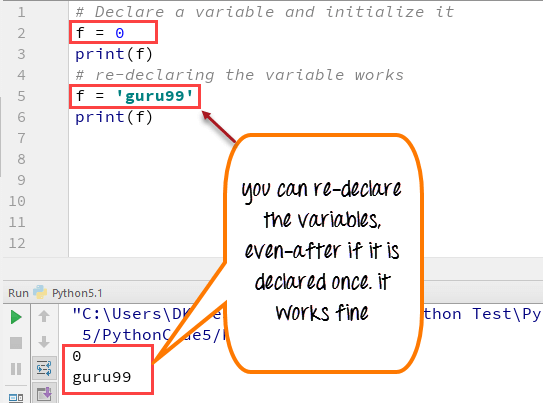Python 2 Example

``````# Declare a variable and initialize it
f = 0
print f
# re-declaring the variable works
f = 'guru99'
print f``````

Python 3 Example

``````# Declare a variable and initialize it
f = 0
print(f)
# re-declaring the variable works
f = 'guru99'
print(f)``````

## Python String Concatenation and Variable

Let’s see whether you can concatenate different data types like string and number together. For example, we will concatenate “Guru” with the number “99”.

Unlike Java, which concatenates number with string without declaring number as string, while declaring variables in Python requires declaring the number as string otherwise it will show a TypeError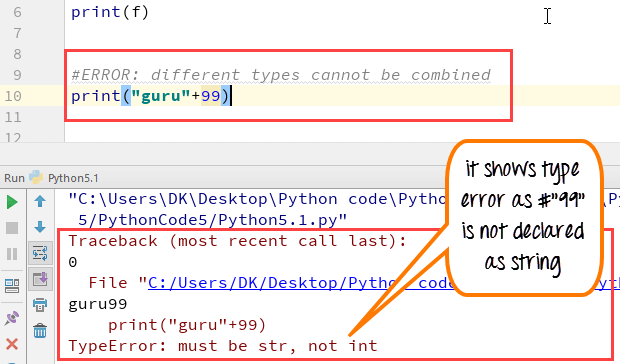For the following code, you will get undefined output –

``````a="Guru"
b = 99
print a+b``````

Once the integer is declared as string, it can concatenate both “Guru” + str(“99”)= “Guru99” in the output.

``````a="Guru"
b = 99
print(a+str(b))``````

## Python Variable Types: Local & Global

There are two types of variables in Python, Global variable and Local variable. When you want to use the same variable for rest of your program or module you declare it as a global variable, while if you want to use the variable in a specific function or method, you use a local variable while Python variable declaration.

Let’s understand this Python variable types with the difference between local and global variables in the below program.

1. Let us define variable in Python where the variable “f” is global in scope and is assigned value 101 which is printed in output
2. Variable f is again declared in function and assumes local scope. It is assigned value “I am learning Python.” which is printed out as an output. This Python declare variable is different from the global variable “f” defined earlier
3. Once the function call is over, the local variable f is destroyed. At line 12, when we again, print the value of “f” is it displays the value of global variable f=101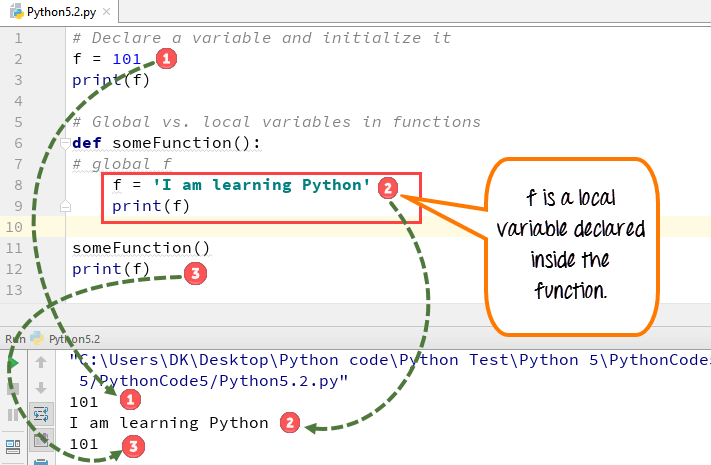Python 2 Example

``````# Declare a variable and initialize it
f = 101
print f
# Global vs. local variables in functions
def someFunction():
# global f
f = 'I am learning Python'
print f
someFunction()
print f``````

Python 3 Example

``````# Declare a variable and initialize it
f = 101
print(f)
# Global vs. local variables in functions
def someFunction():
# global f
f = 'I am learning Python'
print(f)
someFunction()
print(f)``````

While Python variable declaration using the keyword global, you can reference the global variable inside a function.

1. Variable “f” is global in scope and is assigned value 101 which is printed in output
2. Variable f is declared using the keyword global. This is NOT a local variable, but the same global variable declared earlier. Hence when we print its value, the output is 101

We changed the value of “f” inside the function. Once the function call is over, the changed value of the variable “f” persists. At line 12, when we again, print the value of “f” is it displays the value “changing global variable”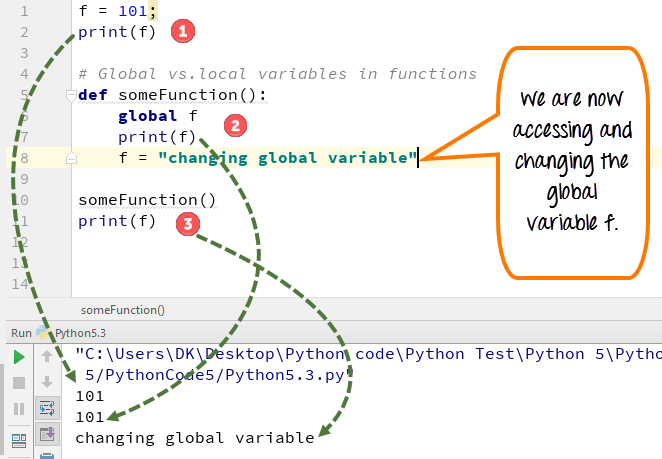Python 2 Example

``````f = 101;
print f
# Global vs.local variables in functions
def someFunction():
global f
print f
f = "changing global variable"
someFunction()
print f``````

Python 3 Example

``````f = 101;
print(f)
# Global vs.local variables in functions
def someFunction():
global f
print(f)
f = "changing global variable"
someFunction()
print(f)``````

## Delete a variable

You can also delete Python variables using the command del “variable name”.

In the below example of Python delete variable, we deleted variable f, and when we proceed to print it, we get error “variable name is not defined” which means you have deleted the variable.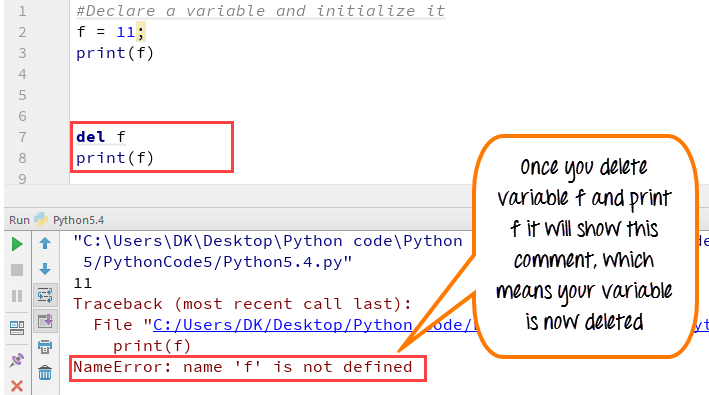Example of Python delete variable or Python clear variable :

``````f = 11;
print(f)
del f
print(f)``````

## Summary:

• Variables are referred to “envelop” or “buckets” where information can be maintained and referenced. Like any other programming language Python also uses a variable to store the information.
• Variables can be declared by any name or even alphabets like a, aa, abc, etc.
• Variables can be re-declared even after you have declared them for once
• Python constants can be understood as types of variables that hold the value which can not be changed. Usually Python constants are referenced from other files. Python define constant is declared in a new or separate file which contains functions, modules, etc.
• Types of variables in Python or Python variable types : Local & Global
• Declare local variable when you want to use it for current function
• Declare Global variable when you want to use the same variable for rest of the program

To delete a variable, it uses keyword “del”.

## A Beginner’s Guide To Python Variables

A variable is a fundamental concept in any programming language. It is a reserved memory location that stores and manipulates data. This tutorial on Python variables will help you learn more about what they are, the different data types of variables, the rules for naming variables in Python. You will also perform some basic operations on numbers and strings. We’ll use Jupyter Notebook to implement the Python codes.

Variables are entities of a program that holds a value. Here is an example of a variable:

x=100

In the below diagram, the box holds a value of 100 and is named as x. Therefore, the variable is x, and the data it holds is the value.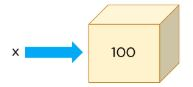The data type for a variable is the type of data it holds.

In the above example, x is holding 100, which is a number, and the data type of x is a number.

In Python, there are three types of numbers: Integer, Float, and Complex.

Integers are numbers without decimal points. Floats are numbers with decimal points. Complex numbers have real parts and imaginary parts.

Another data type that is very different from a number is called a string, which is a collection of characters.

Let’s see a variable with an integer data type:

``x=100``

To check the data type of x, use the type() function:

``type(x)``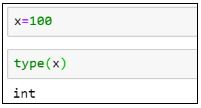Python allows you to assign variables while performing arithmetic operations.

``````x=654*6734
type(x)``````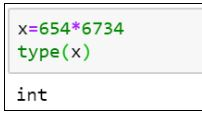To display the output of the variable, use the print() function.

``print(x) #It gives the product of the two numbers``

Now, let’s see an example of a floating-point number:

``````x=3.14
print(x)``````

type(x) #Here the type the variable is float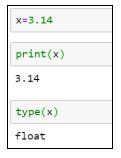Strings are declared within a single or double quote.

``````x=’Simplilearn’

print(x)

x=” Simplilearn.”

print(x)

type(x)``````

In all of the examples above, we only assigned a single value to the variables. Python has specific data types or objects that hold a collection of values, too. A Python List is one such example.

Here is an example of a list:

``````x=[14,67,9]

print(x)

type(x)``````

You can extract the values from the list using the index position method. In lists, the first element index position starts at zero, the second element at one, the third element at two, and so on.

To extract the first element from the list x:

``print(x)``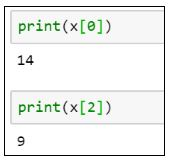To extract the third element from the list x:

``print(x)``

Lists are mutable objects, which means you can change the values in a list once they are declared.

``````x=70 #Reassigning the third element in the list to 70

print(x)``````

Earlier, the elements in the list had [14, 67, 9]. Now, they have [14, 67, 70].

Tuples are a type of Python object that holds a collection of value, which is ordered and immutable. Unlike a list that uses a square bracket, tuples use parentheses.

``````x=(4,8,6)

print(x)

type(x)``````

Similar to lists, tuples can also be extracted with the index position method.

``print(x) #Give the element present at index 1, i.e. 8``

If you want to change any value in a tuple, it will throw an error. Once you have stored the values in a variable for a tuple, it remains the same.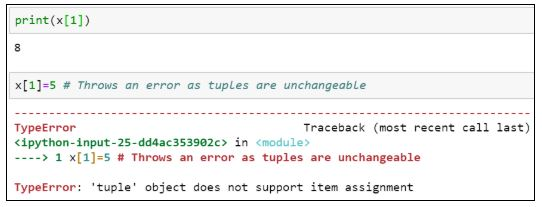When we deal with files, we need a variable that points to it, called file pointers. The advantage of having file pointers is that when you need to perform various operations on a file, instead of providing the file’s entire path location or name every time, you can assign it to a particular variable and use that instead.

Here is how you can assign a variable to a file:

``````x=open(‘C:/Users/Simplilearn/Downloads/JupyterNotebook.ipynb’,’r’)

type(x)``````

Suppose you want to assign values to multiple variables. Instead of having multiple lines of code for each variable, you can assign it in a single line of code.

``(x, y, z)=5, 10, 5``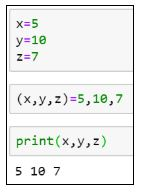The following line code results in an error because the number of values assigned doesn’t match with the number of variables declared.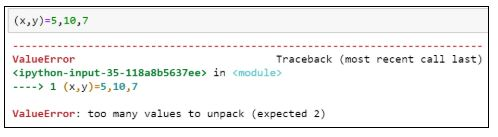If you want to assign the same value to multiple variables, use the following syntax:

``x=y=z=1``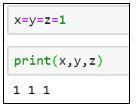Now, let's look at the various rules for naming a variable.

1. A variable name must begin with a letter of the alphabet or an underscore(_)

Example:

``````abc=100 #valid syntax

_abc=100 #valid syntax

3a=10 #invalid syntax

@abc=10 #invalid syntax``````

. The first character can be followed by letters, numbers or underscores.

Example:

``````a100=100 #valid

_a984_=100 #valid

a9967\$=100 #invalid

xyz-2=100 #invalid``````

Python variable names are case sensitive.

Example:

``````a100 is different from A100.

a100=100

A100=200``````

Reserved words cannot be used as variable names.

Example:

``````break, class, try, continue, while, if

break=10

class=5

try=100``````

Python is more effective and more comfortable to perform when you use arithmetic operations.

The following is an example of adding the values of two variables and storing them in a third variable:

``````x=20

y=10

result=x+y

print(result)``````

Similarly, we can perform subtraction as well.

``````result=x-y

print(result)``````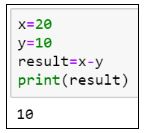Additionally, to perform multiplication and division, try the following lines of code:

``````result=x*y

print(result)

result=x/y

print(result)``````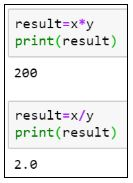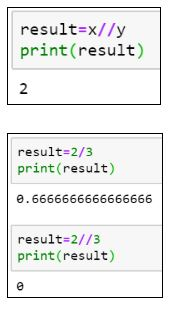As you can see, in the case of division, the result is not an integer, but a float value. To get the result of the division in integers, use “//”the integer division.

The division of two numbers gives you the quotient. To get the remainder, use the modulo (%) operator.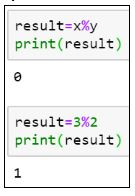Now that we know how to perform arithmetic operations on numbers let us look at some operations that can be performed on string variables.

``var = ‘Simplilearn’``

You can extract each character from the variable using the index position. Similar to lists and tuples, the first element position starts at index zero, the second element index at one, and so on.

``````print(var) #Gives the character at index 0, i.e. S

print(var) #Gives the character at index 4, i.e. l``````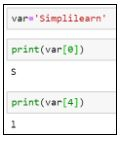If you want to extract a range of characters from the string variable, you can use a colon (:) and provide the range between the ones you want to receive values from. The last index is always excluded. Therefore, you should always provide one plus the number of characters you want to fetch.

``print(var[0:3]) #This will extract the first three characters from zero, first, and second index.``

The same operation can be performed by excluding the starting index.

``print(var[:3])``The following example prints the values from the fifth location until the end of the string.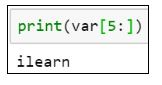Let’s see what happens when you try to print the following:

``print(var[0:20]) #Prints the entire string, although the string does not have 20 characters.``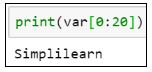To print the length of a string, use the len() function.

``len(var)``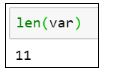Let’s see how you can extract characters from two strings and generate a new string.

``````var1 = “It’s Sunday”

var2 = “Have a great day”``````

The new string should say, “It’s a great Sunday” and be stored in var3.

``````var3 = var1[:5] + var2[5:13] + var1[5:]

print(var3)``````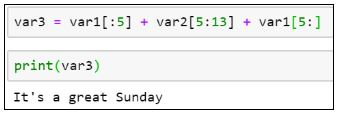Get prepared for your next career as a professional Python programmer with the Python Certification Training Course. Click to enroll now!

## Conclusion

I hope this blog helped you learn the concepts of Python variables. After reading this blog, you may have learned more about what a variable is, rules for declaring a variable, how to perform arithmetic operations on variables, and how to extract elements from numeric and string variables using the index position.

#python #programming1617973320

## ValueError: invalid literal for int() with base 10: ''

Python throws the error, ValueError: invalid literal for int() with base 10:”, when you pass a float string in `int()` function. Although, you can pass the string in `float()` function.

Consider this example –

``````>>> int('5555.00')
Traceback (most recent call last):
File "<stdin>", line 1, in <module>
ValueError: invalid literal for int() with base 10: '5555.00'
``````

PythonCopy

The problem with this code is that you are passing a float string `'5555.00'` in int() function. The correct way of doing this is to first convert it into `float()` and then into `int()`.

#python #error #python error #python-short

1593629492

## Recent Posts - Kodlogs

We provide the latest news and articles on advanced software development topics. Our aim to help solve coding problems, develops new skills, and finds job opportunities. For more details, visit our website.

community problems and solution in development

#valueerror invalid literal for int with base 10 #online php programs in community development #sharing problems and answers as community development #online php programming test questions and answers